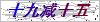# 山东济宁热电阻温度传感器免费咨询：86-0537-3624256发邮件给我们：jnhtck@163.com

接触式温度传感器的检测部分与被测对象有良好的接触，又称温度计。

 HT-T01 螺纹固定式温度传感器选型 铂热电阻类型 P口 0=Pt100     1=Pt1000       5=Pt500     6=Pt10　      9=指定 温度范围(℃) T口 0=0～80  1=-50～100  2=-50～150  3=-50～200    4=-50～300   9=指定 传感器精度 口 1=1级      B=B级 保护管材质 C口 1=SUS321   4=SUS304    6=SUS316       9=指定 保护管直径 D口 5=5  6=6  8=8   12=12  9=指定（说明：温度250℃以上时，保护管直径≥10mm） 螺纹规格 M口 1=M8×1      2=M8×1.25   3=M10×1     4=M10×1.5   5=M12×1 6=M12×1.5   7=M16×1.5   9=其它螺纹规格指定 探头插深长度(mm) G口 40=40  50=50  60=60   80=80  100=100   9= 指定 引线长度(mm) L口 1=100   2=200   3=300   5=500  10=1000  30=3000  50=5000  9=指定 引出线采用线制 X口 2=两线制  3=三线制  4=四线制 引线材质 口 1=聚氯乙烯PVC（-20～80℃）   分有屏蔽层和无屏蔽层两种2=硅橡胶     （-60～150℃）  无屏蔽层3=四氟绕包   （-50～250℃）  有屏蔽层4=云母绕包   （-80～500℃）  无屏蔽层          9=指定 是否配屏蔽层 口 0=无屏蔽层（默认） 1=有屏蔽层 导线末端要求 口 0=无   1=镀锡  2=U型端子  3=针式端子 9=指定 特殊要求 Q口 0=无    1=配套管    9=指定 注意事项：1、选用云母绕包线时，插深长度≥50mm，配其它线时，插深长度≥40mm，特殊情况定制。                   2、选用云母绕包线时，螺纹部分直径≥16mm ，配其它线缆时，螺纹部分直径≥8mm ，特殊情况定制。

 HT-T02 螺纹固定式数字温度传感器选型 传感器类型 D 数字温度传感器DS18B20    （有关数字DS18B20介绍请见第4页相关内容） 温度范围(℃) T口 0=－20～80    1=-55～125 保护管材质 C口 1=SUS321   4=SUS304    6=SUS316       9=指定 保护管直径 D口 6=6     8=8      12=12       9=指定 螺纹规格 M口 1=M10×1  2=M10×1.5   3=M12×1  4=M12×1.5   5=M16×1.5   9=指定 探头插深长度(mm) G口 40=40   50=50　 60=60   80=80  100=100  150=150     9=指定 引线长度(mm) L口 1=100   2=200   3=300   5=500  10=1000  30=3000  50=5000  9=指定 引线材质 口 1=聚氯乙烯PVC（-20～80℃）   2=硅橡胶（-60～150℃）无屏蔽层 是否配屏蔽层 口 0=无屏蔽层（默认）    1=有屏蔽层 导线末端要求 口 0=无   1=镀锡    2=U型端子   3=针式端子   9=指定 特殊要求 Q口 0=无     1=配套管     9=指定

### 留言框

• #### 验证码：请输入计算结果（填写阿拉伯数字），如：三加四=7

© 2021 版权所有：济宁恒通仪表测控技术有限公司  备案号：  总访问量：172650  站点地图  技术支持：仪表网  管理登陆

QQ在线客服

15763783659

86-0537-3624256